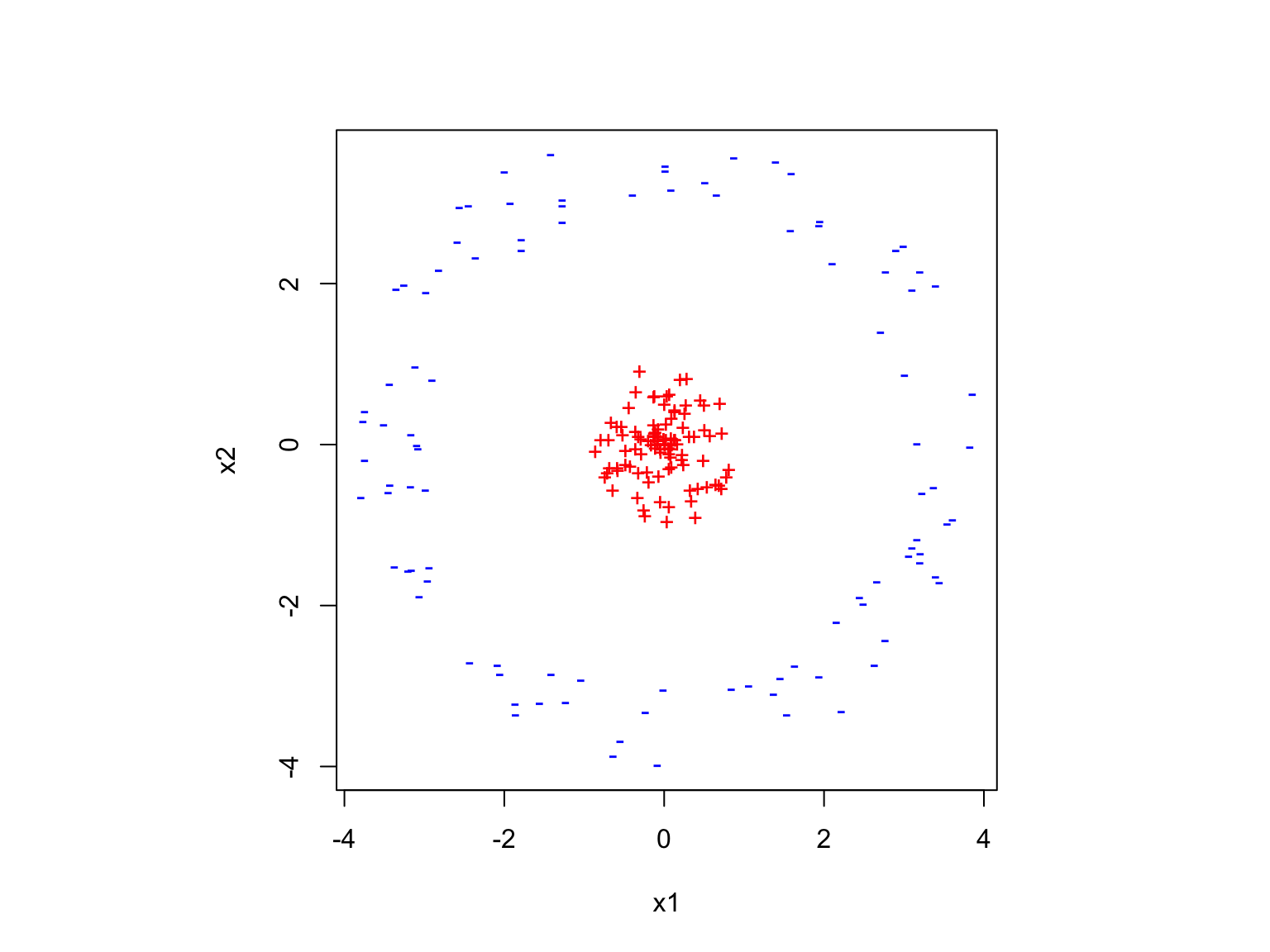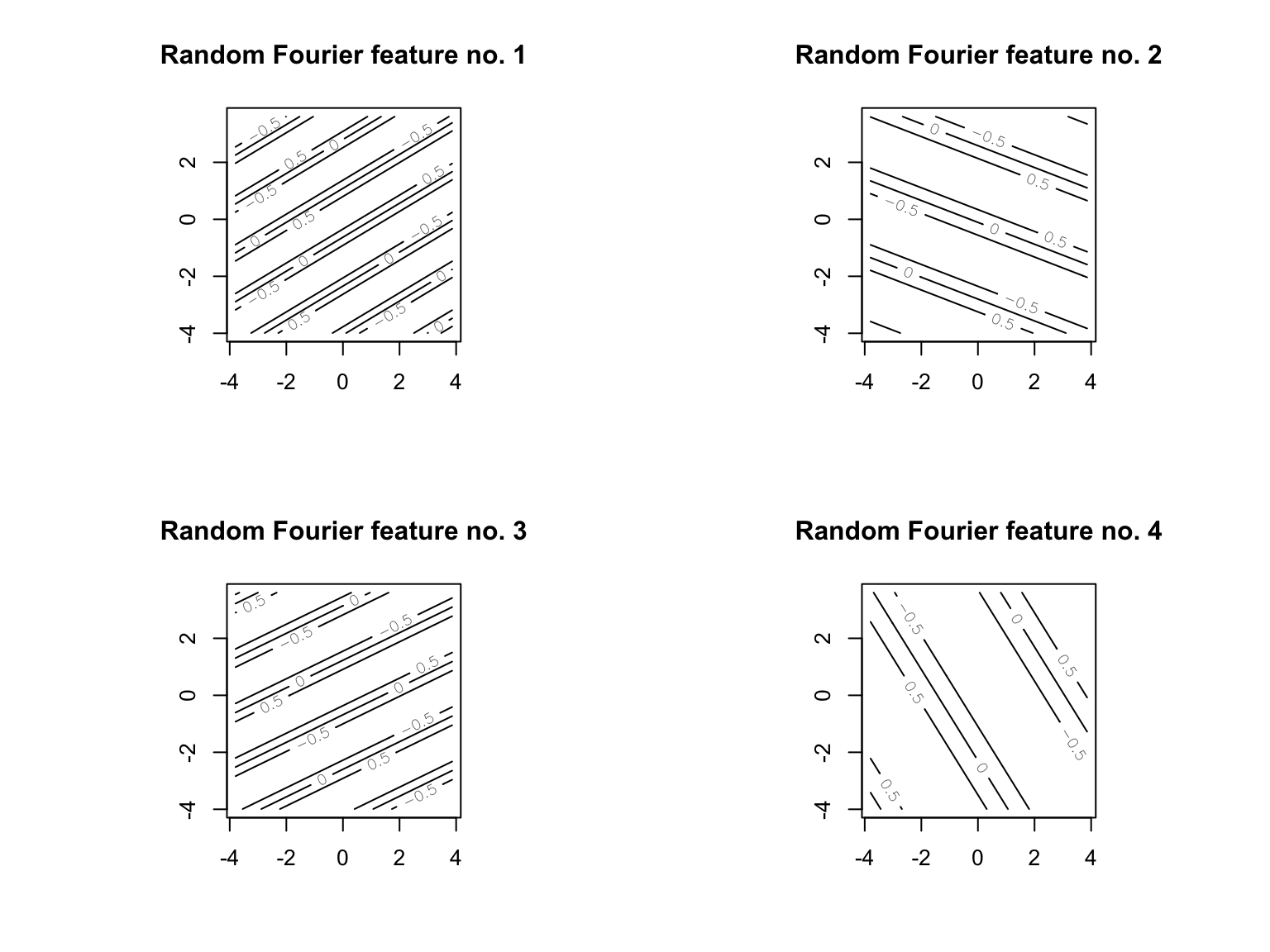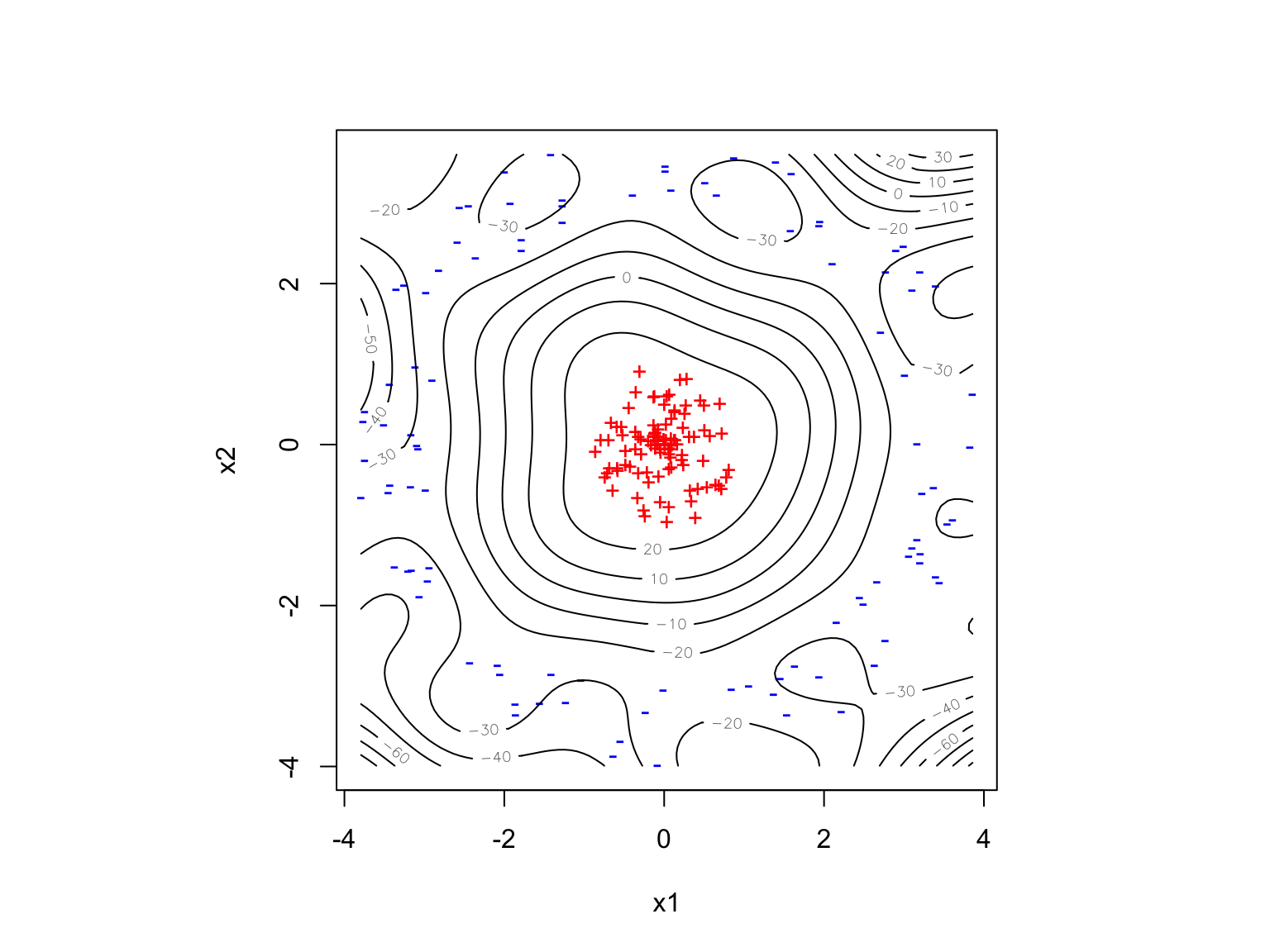# Random Feature Machines, a.k.a. Random Kitchen Sinks

22 April 2021 (Lecture 22)

$\newcommand{\Prob}{\mathbb{P}\left( #1 \right)} \newcommand{\Expect}{\mathbb{E}\left[ #1 \right]} \newcommand{\Var}{\mathrm{Var}\left[ #1 \right]} \newcommand{\Cov}{\mathrm{Cov}\left[ #1 \right]} \newcommand{\Risk}{r} \newcommand{\EmpRisk}{\hat{\Risk}} \newcommand{\Loss}{\ell} \newcommand{\OptimalStrategy}{\sigma} \DeclareMathOperator*{\argmin}{argmin} \newcommand{\ModelClass}{S} \newcommand{\OptimalModel}{s^*} \DeclareMathOperator{\tr}{tr} \newcommand{\Indicator}{\mathbb{1}\left\{ #1 \right\}} \newcommand{\myexp}{\exp{\left( #1 \right)}} \newcommand{\eqdist}{\stackrel{d}{=}} \newcommand{\Rademacher}{\mathcal{R}} \newcommand{\EmpRademacher}{\hat{\Rademacher}} \newcommand{\Growth}{\Pi} \newcommand{\VCD}{\mathrm{VCdim}} \newcommand{\OptDomain}{\Theta} \newcommand{\OptDim}{p} \newcommand{\optimand}{\theta} \newcommand{\altoptimand}{\optimand^{\prime}} \newcommand{\ObjFunc}{M} \newcommand{\outputoptimand}{\optimand_{\mathrm{out}}} \newcommand{\Hessian}{\mathbf{h}} \newcommand{\Penalty}{\Omega} \newcommand{\Lagrangian}{\mathcal{L}} \newcommand{\HoldoutRisk}{\tilde{\Risk}} \DeclareMathOperator{\sgn}{sgn} \newcommand{\Margin}{M}$

# Reminders

• Kernel machines: $s(x) = b_0 + \sum_{i=1}^{n}{\alpha_i K(x, x_i)} = b_0 + \sum_{j=1}^{\infty}{\beta_j \phi_j(x)} = b_0 + \langle \beta, \phi(x) \rangle$
• Kernel matrix $$\mathbf{K} =$$ the $$[n\times n]$$ matrix where $$K_{ij} = K(x_i, x_j)$$
• If $$\| \beta \| \leq c$$ then (HW10Q1) $\EmpRademacher_n(\ModelClass) \leq \frac{c\sqrt{\tr{\mathbf{K}}}}{n}$
• And (HW10Q2) $$\|\beta\|^2 = \alpha \cdot \mathbf{K} \alpha$$

# Reminders (2)

• Regression: use kernel ridge regression, $\min_{\beta}{\frac{1}{n}\sum_{i=1}^{n}{(y_i - s(x_i;\beta))^2} + \lambda \|\beta\|^2} = \min_{\alpha \in \mathbb{R}^n}{\frac{1}{n}\sum_{i=1}^{n}{(y_i - s(x_i;\alpha))^2} + \lambda \left(\alpha \cdot \mathbf{K} \alpha\right)}$
• Solution: $$\alpha = (\mathbf{K} + \lambda \mathbf{I})^{-1} \mathbf{y}$$
• Great for least-squares regression, awkward for other tasks
• Classification: classes $$y_i$$ are $$\pm 1$$, maximize the margin by minimizing $\sum_{i=1}^{n}{\sum_{j=1}^{n}{\alpha_i \alpha_j y_i y_j K(x_i, x_j)}}$ subject to $$\sum_{i=1}^{n}{\alpha_i y_i} = 0$$, $$\sum_{i=1}^{n}{\alpha_i}=1$$, $$\alpha_i \geq 0$$
• Slightly different objective if we allow for some slack/mis-classification
• Solution: support vector machine: $\sgn{\left(b_0 + \sum_{i=1}^{n}{\alpha_i y_i K(x, x_i)} \right)}$
• Many/most $$\alpha_i = 0$$ (sparse), if $$\alpha_i \neq 0$$ then $$x_i$$ is a support vector

# Kernel machines are great

• The space of functions of the form $$\sum_{j=1}^{\infty}{\beta_j \phi_j(x)}$$ is huge!
• Not every function, but lots of functions, and even if the best function isn’t in this space, it can often be closely approximated
• But we only have to find the $$n$$ weights $$\alpha_i$$
• Finite-dimensional approximations to an infinite-dimensional space
• Kernels measure similarity and there are lots of good ways to do that
• Radial basis functions, polynomials, splines…
• Specialized kernels for strings (think: text, DNA), wave-forms (think: speech, music), images, graphs/networks, …
• Learning-theoretic results so there are both generalization error bounds and oracle inequalities
• We can make guarantees about out-of-sample performance
• We can make guarantees about near-optimality
• Kernel machines became big around 1995 with the first support vector machines, and pretty much ruled until say 2012 or 2013
• Deep neural networks trained by gradient descent may be kernel machines in disguise (Domingos 2020)

# Kernel machines are not so great

• Need to think hard about the kernel function $$K$$
• Computationally, everything comes down to the kernel matrix $$\mathbf{K}$$
• $$\mathbf{K}$$ is $$[n\times n]$$
• Not so bad when $$n=10^2$$ or $$10^3$$ or $$10^4$$
• Awkward when $$n=10^6$$ (8 Tb of memory just to store one matrix!)
• Inverting a matrix takes $$O(n^3)$$ time, but even just calculating the objective function for an SVM takes $$O(n^2)$$ time
• Very elaborate algorithms can invert in $$O(n^{2.37\ldots})$$ time (but can actually be slower at small $$n$$
• Also, you need to keep around all $$n$$ weights $$\alpha_1, \ldots \alpha_n$$
• Even with SVMs, the number of support vectors is typically $$\propto n$$

# Random feature machines, a.k.a. kitchen sinks

• Come up with a big collection of basis functions, say $$\psi(x;w)$$, where the $$w$$ are indices or parameters
• Example: $$\psi(x;w) = \cos{(w_1 \cdot x + w_2)}$$ (cosine basis or Fourier basis)
• Example: $$\psi(x;w) = \Indicator{\|x - w\| \leq h}$$ (bumps)
• Randomly draw values $$W_1, W_2, \ldots W_q$$ from some distribution $$\rho$$ over $$w$$
• Now fit $\sum_{j=1}^{q}{\beta_j \psi(x;W_j)}$

# What does this have to do with kernels?

Bochner’s theorem: If $$K(x,z)=K(x-z)$$ is a kernel over $$\mathbb{R}^d$$, then there’s a probability distribution $$\rho$$ over $$\mathbb{R}^d$$ and a constant $$C>0$$ where $K(x,z) = C \int_{\mathbb{R}^d}{\rho(w)\cos{(w\cdot(x-z))} dw}$ + Note: $$K(x,z)/C$$ is another kernel with the same feature space, so we can set $$C=1$$ “without loss of generality”

• $$\rho$$ is the Fourier transform of $$K(x-z)$$
• Implication: If $$W \sim \rho$$, then $K(x,z) = \Expect{\cos{(W \cdot (x-z))}}$
• Implication: if $$W_1, W_2, \ldots W_q$$ are all $$\sim \rho$$, then $\frac{1}{q}\sum_{j=1}^{q}{\cos{(W_j \cdot(x-z))}} \approx \Expect{\cos{(W \cdot (x-z))}} = K(x,z)$

# What does this have to do with kernels? (2)

• Remember $$K(x,z) = \langle \phi(x), \phi(z) \rangle$$
• After some play with trig. identities, two sets of random features will work: $\psi_i(x) = (\cos{W_i \cdot x}, \sin{W_i \cdot x})$ or $\psi_i(x) = \cos{W_i \cdot x + b_i}$ where $$b \sim\mathrm{Unif}(-\pi, \pi)$$
• More common to use the 2nd approach (random “phase” $$b_i$$)
• Define $\psi(x) = (\psi_1(x), \psi_2(x), \ldots \psi_q(x))$
• Then $\Expect{\psi(x) \cdot \psi(z)} = K(x,z)$
• Since $$\cos$$ is bounded between $$-1$$ and $$1$$, we can use Hoeffding’s inequality to show $$\psi(x) \cdot \psi(z)$$ is close to $$K(x,z)$$ with high probability like $$e^{-q\epsilon^2}$$
• In fact $$\Prob{\max_{x,z}{|\psi(x) \cdot \psi(z) - K(x,z)| \geq \epsilon}} = O(\epsilon^{-1}e^{-q\epsilon^2})$$ (omitting constants in exponent etc.) (Rahimi and Recht 2008, claim 1)

# Random feature approximation to kernel machines

• Pick your favorite kernel $$K$$ and a number $$q > 0$$
• Find $$K$$’s Fourier transform $$\rho$$
• People have been tabulating Fourier transforms since Fourier almost 200 years ago, you can usually look it up
• Conveniently, the Fourier transform of a Gaussian is also a Gaussian
• Draw $$W_1, W_2, \ldots W_q$$ from $$\rho$$ and $$b_1, b_2, \ldots b_q$$ from $$\mathrm{Unif}(-\pi, \pi)$$
• Calculate the random Fourier features of the data $\psi(x) = (\cos{W_1 \cdot x + b_1}, \cos{W_2 \cdot x +b_2}, \ldots \cos{W_q \cdot x + b_q})$
• Form the $$[n \times q]$$ matrix $$\mathbf{\psi}$$
• Now run your favorite linear method on $$\mathbf{\psi}$$
• $$\mathbf{\psi}\mathbf{\psi}^T \approx \mathbf{K}$$, a low-rank (rank-$$q$$) approximation, and there can be situations where we want to use that because even though it’s $$[n \times n]$$ there are special computational techniques for low-rank matrices; more common however to just work with $$\mathbf{\psi}$$

# We don’t really need a kernel

• The random kitchen sink idea of Rahimi and Recht (2009)
• Pick your favorite parameterized collection of functions $$\psi(x;w)$$, a distribution $$\rho(w)$$, an integer $$q$$, and a $$C> 0$$
• Draw $$W_1, \ldots W_q$$ from $$\rho$$
• Calculate $$\psi(x_i) = (\psi(x_i;W_1), \ldots \psi(x_i, W_q))$$
• Do constrained ERM over linear models in these features: $\hat{\beta} = \argmin_{\beta: \max_{i \in 1:q}{|\beta_i| \leq C/q}}{\frac{1}{n}\sum_{i=1}^{n}{\Loss(y_i, \beta \cdot \psi(x_i))}}$
• If the loss function $$\Loss$$ is reasonable, then we have an oracle inequality: $\Prob{\Risk(\hat{s}) - \Risk(\OptimalModel) \geq O\left(\left(\frac{1}{\sqrt{n}}+\frac{1}{\sqrt{q}}\right) C \sqrt{\log{(2/\delta)}}\right)} \leq \delta$
• You’ll work this through in HW13, with all the constants
• Basic idea: The random features give a good approximation to the optimal machine (that’s where the $$O(1/\sqrt{q})$$ comes from), and linear models on the random features have low Rademacher complexity (that’s where the $$O(1/\sqrt{n})$$ comes from)

# Kitchen sinks in practice

• Want the function space to be rich
• Random Fourier features are good, so are random bumps
• Typically make $$C$$ large enough that the constraint stays inactive
• Or just ignore it altogether…
• Can also do ridge or lasso, or make the linear part the input to a generalized linear model, etc.

# A demo in Rlibrary(expandFunctions)
my.rFfs <- raptMake(p = 2, q = 30, WdistOpt = list(sd = 1), bDistOpt = list(min = -pi,
max = pi))
dim(rapt(as.matrix(df[, c("x1", "x2")]), my.rFfs))
##  200  30
df.augmented <- data.frame(y = df$y, cos(rapt(as.matrix(df[, c("x1", "x2")]), my.rFfs))) Here are the first few $$W_j$$ ## [,1] [,2] ## [1,] 1.0917135 -1.83151364 ## [2,] 0.4475455 1.16703196 ## [3,] 0.7942617 -1.64220647 ## [4,] -0.6990022 -0.43673732 ## [5,] -1.3940621 -0.48644337 ## [6,] 0.9985419 -0.09824253 and the corresponding $$b_j$$ ## [,1] ## [1,] 0.4192803 ## [2,] -1.4453380 ## [3,] 0.4489669 ## [4,] -2.5730839 ## [5,] -2.2053458 ## [6,] 0.4401365• The features have random orientations, wavelengths, and phase off-sets • Any model we build is going to be a linear combination of these random cosine waves glm.rks <- glm(y ~ ., data = df.augmented, family = "binomial") table(predict(glm.rks, type = "response") >= 0.5, df.augmented$y)
##
##           0   1
##   FALSE 100   0
##   TRUE    0 100# Summing up

• Kernel machines are great in many ways
• but there are computational bottlenecks for large $$n$$
• Every prediction needs $$O(n)$$ calculations and requires remembering $$O(n)$$ weights $$\alpha_i$$
• The $$[n \times n]$$ kernel matrix $$\mathbf{K}$$ is needs lots of memory and time
• Random feature machines emerged as an approximation to kernel machines
• Each prediction needs $$O(q)$$ calculations and requires remembering $$q$$ weights $$\beta_j$$
• Working with linear-in-the-features methods needs only $$[q\times q]$$ matrices
• Random feature machines turned out to be useful in their own right, not just as approximations to kernel machines
• Very simple computationally (especially if you use random Fourier features)
• Highly expressive with not-too-many random features
• Good generalization properties
• Good in-practice performance